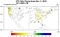# Working with geospatial data in PythonCPC Unified Gauge-Based Analysis of Daily Precipitation over CONUS
`conda create -n env_name python=3.6source activate env_name`
`python -m ipykernel install user --name env --name --display-name “Name to Show in Jupyter”`
`def get_CPC_precip_lat_lon(lat,lon,yr_range):  grid_precip_ts = pd.Series()  for yr in yr_range:  fname = 'path_to_directory/precip.'+str(yr)+'.nc'  ds = xr.open_dataset(fname)  df = ds.to_dataframe()  yr_ts = df.loc[(lat,lon)]  yr_ts.index.name = None   yr_ts = pd.Series(yr_ts.values.squeeze(),index=yr_ts.index.values)  grid_precip_ts = pd.concat([grid_precip_ts,yr_ts],axis=0)  return(grid_precip_ts)`
`#loop through years to process each file#remember to change fname to relevant pathstats = pd.DataFrame()for yr in yr_range:  print("Checking data for: "+str(yr))fname = '/Users/Osho/cpc_noaa/precip.'+str(yr)+'.nc'   ds = xr.open_dataset(fname)  df_data = ds.to_dataframe()missing_days = tabulateMissingDays_general(df_data) missing_days.insert(loc=0,column='year',     value[yr]*len(missing_days))stats = pd.concat([stats, missing_days], axis=0)`
`def tabulateMissingDays_general(df_all_data): df_all_data.reset_index(inplace=True)  #cutting out antarctica and arctic trim_locations = df_all_data[(df_all_data['lat']>=-60) & (df_all_data['lat']<=80)].copy(deep=True) trim_locations['isNaN'] = np.isnan(trim_locations['precip'])  unique_locations = trim_locations[['lat','lon']].drop_duplicates(subset=['lat', 'lon'], keep='first') total_measurements = len(unique_locations)  nan_rows = trim_locations[trim_locations['isNaN']==True]  #you can do alot of different subsets to get more details on the specific lat, lon #and the specific days. this approach is best for general data checkingcount_nan = nan_rows[['lat','lon','time']] count_nan = count_nan.groupby(['lat', 'lon']).agg({'time':np.size}) count_nan.columns = ['missing_days']  ctr = collections.Counter(count_nan['missing_days']) unique_nans = pd.DataFrame.from_dict(ctr, orient='index').reset_index() unique_nans.columns = ['missing_days', 'num_locations'] unique_nans['missing_locations_percent'] = unique_nans['num_locations']/total_measurements unique_nans['missing_locations_percent'] = pd.Series(["{0:.2f}%".format(val * 100)  for val in unique_nans['missing_locations_percent']], index = unique_nans.index)  return(unique_nans)`
`#remember to edit the function name in the loop we created abovedef tabulateMissingDays_locations(df_all_data): df_all_data.reset_index(inplace=True)  #cutting out antarctica and arctic trim_locations = df_all_data[(df_all_data['lat']>=-60) & (df_all_data['lat']<=80)].copy(deep=True) trim_locations['isNaN'] = np.isnan(trim_locations['precip'])  nan_rows = trim_locations[trim_locations['isNaN']==True]  count_nan = nan_rows[['lat','lon','time']] count_nan = count_nan.groupby(['lat', 'lon']).agg({'time':np.size}) count_nan.columns = ['missing_days']  return(count_nan)`
`shape_file_dir = "path_to_shapefile_directory"def getShapeFiles(directory):os.chdir(directory)file_list = []for file in glob.glob("*.shp"):  file_list.append(directory+"/"+file)return(file_list)shape_files = getShapeFiles(shape_file_dir)gdf_shape_files = []for file in shape_files:  gdf_shape_files.append(gpd.read_file((file)))gdf_all_geos = pd.concat(gdf_shape_files, sort=False)`
`geometry = unique_locations.apply(lambda x:Point([x['lat'],x['lon']]),axis=1)unique_locations["geometry"]= geometryunique_locations_gdp = gpd.GeoDataFrame(unique_locations,geometry=geometry)unique_locations_gdp.crs = {"init" :"epsg:4326"}tagged_locations = gpd.sjoin(unique_locations_gdp, global_data, op="within")`

Written by

## Arbol

#### A weather immunity marketplace powered by blockchain Learn more at https://arbolmarket.com

Welcome to a place where words matter. On Medium, smart voices and original ideas take center stage - with no ads in sight. Watch
Follow all the topics you care about, and we’ll deliver the best stories for you to your homepage and inbox. Explore
Get unlimited access to the best stories on Medium — and support writers while you’re at it. Just \$5/month. Upgrade